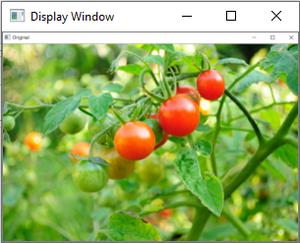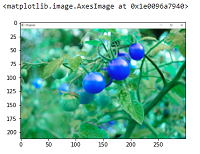GFG App
Open AppBrowser
Continue

# Reading an image in OpenCV using Python

Prerequisite: Basics of OpenCV

In this article, we’ll try to open an image by using OpenCV (Open Source Computer Vision) library.  Following types of files are supported in OpenCV library:

• Windows bitmaps – *.bmp, *.dib
• JPEG files – *.jpeg, *.jpg
• Portable Network Graphics – *.png
• WebP – *.webp
• Sun rasters – *.sr, *.ras
• TIFF files – *.tiff, *.tif
• Raster and Vector geospatial data supported by GDAL

To use the OpenCV library in python, we need to install these libraries as a prerequisite:

1. Numpy Library : The computer processes images in the form of a matrix for which NumPy is used and  OpenCV uses it in the background.
2. OpenCV python : OpenCV library previously it was cv but the updated version is cv2. It is used to manipulate images and videos.

To install these libraries, we need to run these pip commands in cmd:

```pip install opencv-python
pip install numpy
pip install matplotlib```

The steps to read and display an image in OpenCV are:

2. Create a GUI window and display image using imshow() function.

3. Use function waitkey(0) to hold the image window on the screen by the specified number of seconds, o means till the user closes it, it will hold GUI window on the screen.

4. Delete image window from the memory after displaying using destroyAllWindows() function.

Let’s start reading an image. using cv2.
To read the images cv2.imread() method is used. This method loads an image from the specified file. If the image cannot be read (because of missing file, improper permissions, unsupported or invalid format) then this method returns an empty matrix.

Parameters:
path: A string representing the path of the image to be read.
flag: It specifies the way in which image should be read. It’s default value is cv2.IMREAD_COLOR

Return Value: This method returns an image that is loaded from the specified file.

Note:

1. The image should be in the working directory or a full path of image should be given.
2. By default, OpenCV stores colored images in BGR(Blue Green and Red) format.

All three types of flags are described below:

cv2.IMREAD_COLOR: It specifies to load a color image. Any transparency of image will be neglected. It is the default flag. Alternatively, we can pass integer value 1 for this flag.
cv2.IMREAD_GRAYSCALE: It specifies to load an image in grayscale mode. Alternatively, we can pass integer value 0 for this flag.
cv2.IMREAD_UNCHANGED: It specifies to load an image as such including alpha channel. Alternatively, we can pass integer value -1 for this flag.

Below codes are implementations to read images and display images on the screen using OpenCV and matplotlib libraries functions.

Example #1 (Using OpenCV) :

Image used is:## Python3

 `# Python code to read image` `import` `cv2`   `# To read image from disk, we use` `# cv2.imread function, in below method,` `img ``=` `cv2.imread(``"geeksforgeeks.png"``, cv2.IMREAD_COLOR)`   `# Creating GUI window to display an image on screen` `# first Parameter is windows title (should be in string format)` `# Second Parameter is image array` `cv2.imshow(``"image"``, img)`   `# To hold the window on screen, we use cv2.waitKey method` `# Once it detected the close input, it will release the control` `# To the next line` `# First Parameter is for holding screen for specified milliseconds` `# It should be positive integer. If 0 pass an parameter, then it will` `# hold the screen until user close it.` `cv2.waitKey(``0``)`   `# It is for removing/deleting created GUI window from screen` `# and memory` `cv2.destroyAllWindows()`

Output:Reading an image using cv2 in BGR format

We can see the shape , i.e., width and height and channels of the image using shape attribute.

`img.shape`
```Output:
(225, 225, 3)```

Matplotlib library uses RGB color format to read a colored image. Here we are demonstrating an example of reading an image using this library.
Example#2

## Python3

 `#import cv2, numpy and matplotlib libraries` `import` `cv2` `import` `numpy as np` `import` `matplotlib.pyplot as plt` `img``=``cv2.imread(``"geeks.png"``)` `#Displaying image using plt.imshow() method` `plt.imshow(img)`   `#hold the window` `plt.waitforbuttonpress()` `plt.close(``'all'``)`Image read using cv2 and displaying using matplotlib will display picture in BGR format.

Note: See the difference in colors of images read by cv2 and matplotlib library. Because cv2 uses BGR color format and matplotlib uses RGB color format. To convert BGR to RGB, we us a function:

Example#3:

## Python3

 `#import cv2, numpy and matplotlib libraries` `import` `cv2` `import` `numpy as np` `import` `matplotlib.pyplot as plt` `img``=``cv2.imread(``"geeks.png"``)`   `# Converting BGR color to RGB color format` `RGB_img ``=` `cv2.cvtColor(img, cv2.COLOR_BGR2RGB)`   `#Displaying image using plt.imshow() method` `plt.imshow(RGB_img)`   `# hold the window` `plt.waitforbuttonpress()` `plt.close(``'all'``)`

Output:BGR color format changes to RGB color format

Example #4: Opening in grayscale mode

## Python

 `# Python program to explain cv2.imread() method `   `# importing cv2 ` `import` `cv2 `   `# path ` `path ``=` `r``'geeksforgeeks.png'`   `# Using cv2.imread() method ` `# Using 0 to read image in grayscale mode ` `img ``=` `cv2.imread(path, cv2.IMREAD_GRAYSCALE) `   `# Displaying the image ` `cv2.imshow(``'image'``, img) ` `cv2.waitKey(``0``)` `cv2.destroyAllWindows()`

Output :The shape of the grayscale image can be displayed using shape attribute.

`img.shape`
```Output:
(225, 225)```

My Personal Notes arrow_drop_up